# Word2Vec（2013年1月）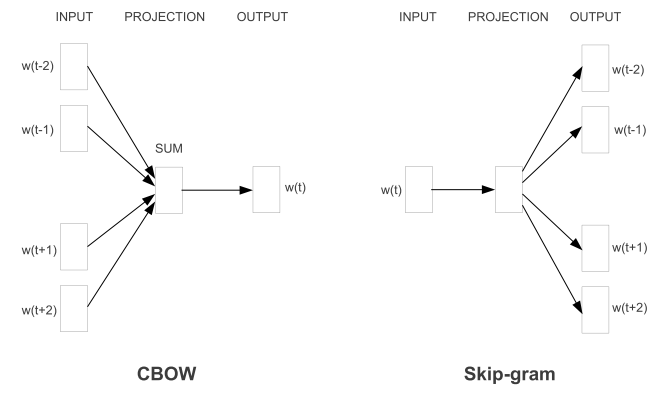CBOW用上下文训练目标词，而Skip-gram用目标词去预测上下文。Word2Vec采用三层神经网络就可以训练，最后一层的输出是Softmax，因为Softmax需要计算全局的token，因此速度慢。为了提高训练速度，衍生出用Huffman树和负采样代替最简单的Softmax的训练方法。

# GloVe（2014年1月）

Global Vectors for Word Representation。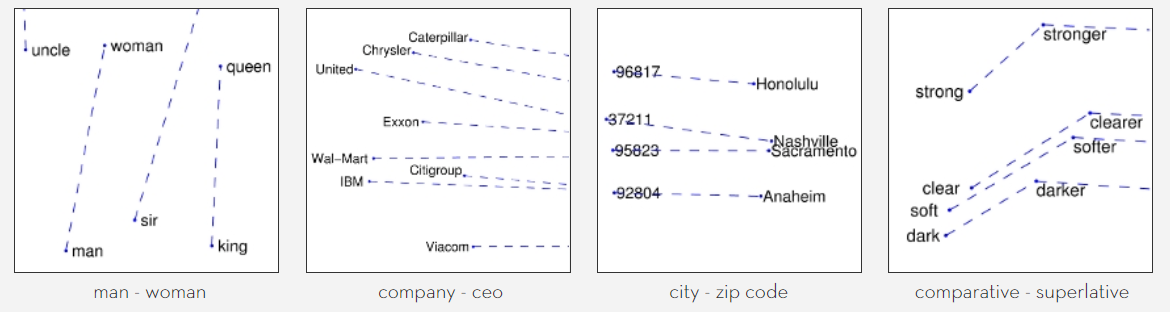Word2Vec的目的是预测，而GloVe的目的是将共现矩阵降维。不过共现矩阵的维度太大不可能直接构造出来用传统方法降维，所以GloVe的工作实际上可以理解为重构共现矩阵。

$P_{ik}/P_{jk}$ 单词j和单词k相关 单词j和单词k不相关

$\frac{P_{i,k}}{P_{j,k}} = g(i,j,k)$

$J = \sum_{i,j,k}(P_{i,k}/P_{j,k} − g(i,j,k))^2$

$g(i,j,k) = exp⁡(v_i^T v_k − v_j^T v_k) = \frac{exp⁡(v_i^T v_k)}{exp⁡(v_j^T v_k)}$

$\frac{P_{i,k}}{P_{j,k}} = \frac{exp⁡(v_i^T v_k)}{exp⁡(v_j^T v_k)}$

$P_{i,k} = exp⁡(v_i^T v_k)$

$log⁡(P_{i,k}) = v_i^T v_k$

$J = \sum_{i,k}(log⁡(P_{i,k}) − v_i^T v_k)^2$

$log⁡(X_{i,k}) = v_i^T v_k − log⁡(X_i)$

$log⁡(X_{i,k}) = v_i^T v_k − log⁡(X_i) + b_k = v_i^T v_k + b_i + b_k$

$J = \sum_{i,k}{v_i^T v_k + b_i + b_k − log⁡(X_{i,k}))^2}$

f(x) = \begin{aligned} \begin{cases} (\frac{x}{xmax})^{0.75}, &if \ x < xmax \\ 1, &if \ x \ge xmax \end{cases} \end{aligned}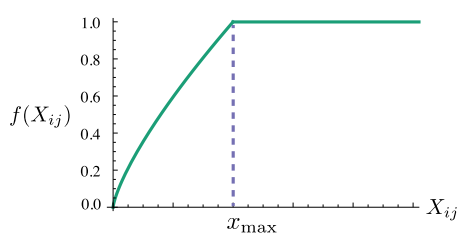$J = \sum_{i,k}{f(X_{i,k})(v_i^T v_k + b_i + b_k − log⁡(X_{i,k}))^2}$

# FastText（2016年7月）

FastText的模型架构和CBOW非常相似，不同之处在于，FastText预测标签，而CBOW模型预测中间词。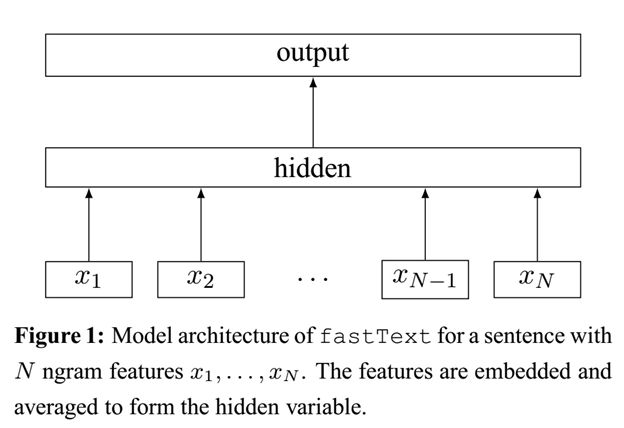FastText有以下特点：

1. 利用了subword特性，比如“English-born”和“China-born”可以共享“born”信息。这对于英语等西语来说是十分有效的，但是放在中文下可能不那么奏效。关于subword的具体实现在“Enriching Word Vectors with Subword Information”中。
2. subword在文本分类任务中的output是文本标签，并且 输入是一篇文章中所有的词
3. 使用了Hierarchy Softmax或Negative sampling加速训练。
4. 融合Ngram特性。因为FastText将整篇文章的所有单词作为输入，并且hidden层是所有词的平均，所以词序变得不那么重要了。为了解决解决这个问题，FastText将每一个n-gram看成一个词，在计算hidden向量的时候把这些n-gram也加进去。但是n-gram的数量是非常大的，完全存下这些embedding也不现实，因此作者把这些n-gram的哈希到N个桶中，同一个桶中的所有n-gram共享同一个embedding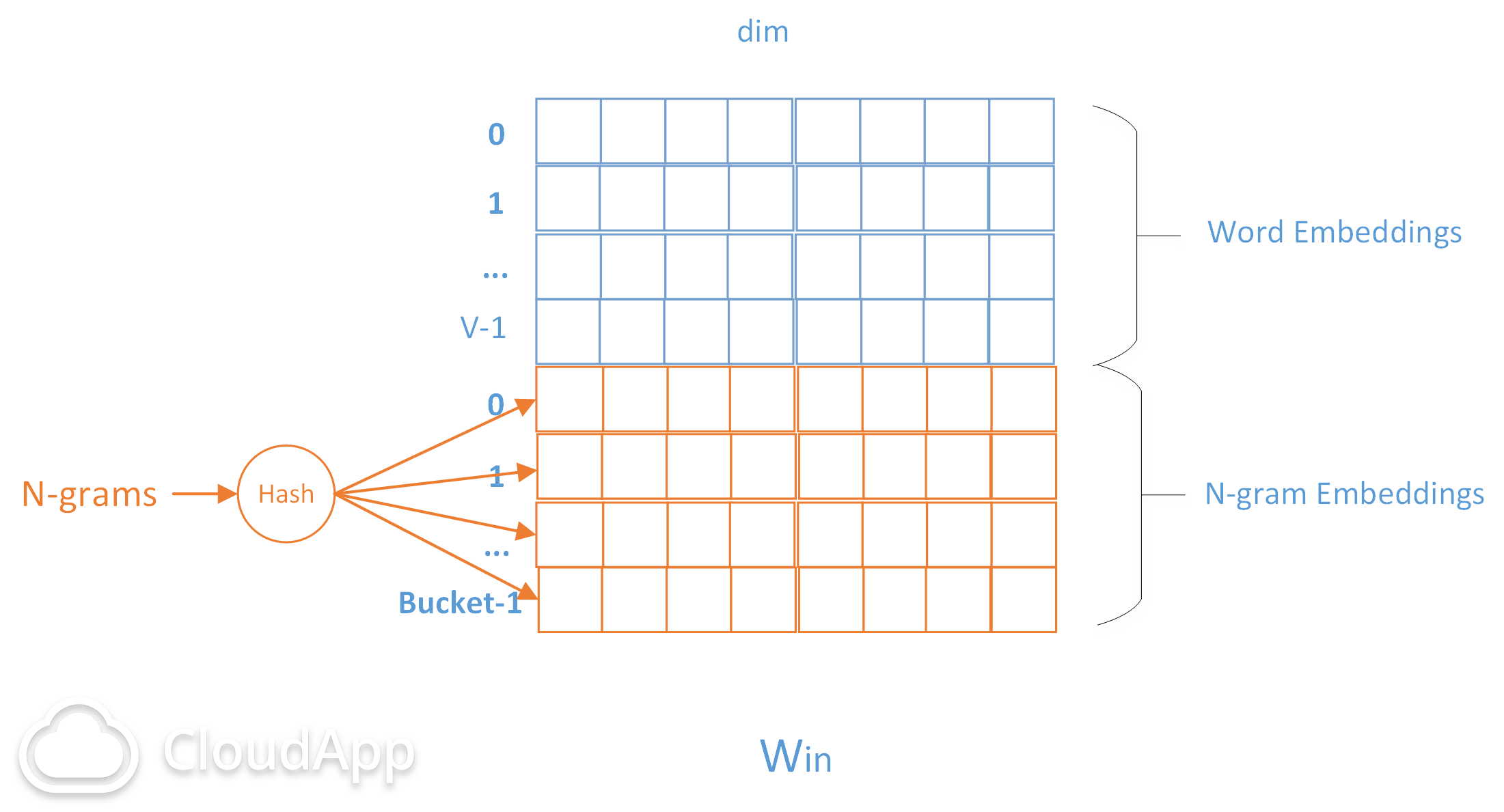# Transformer（2017年6月）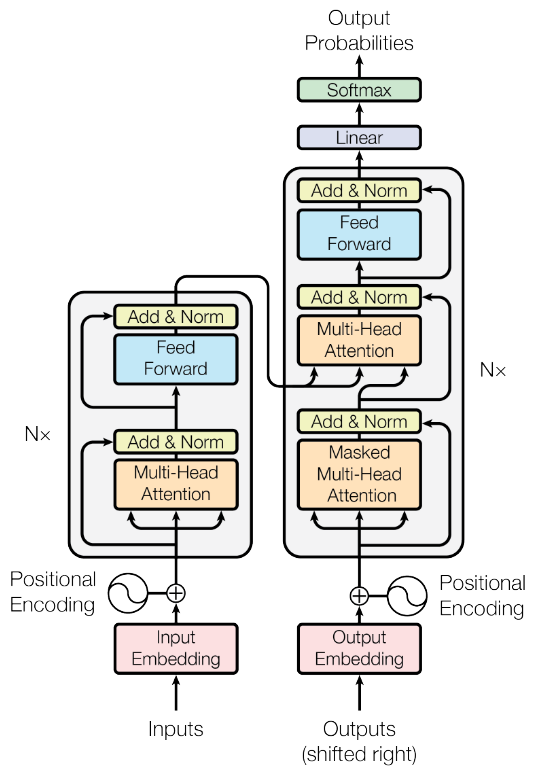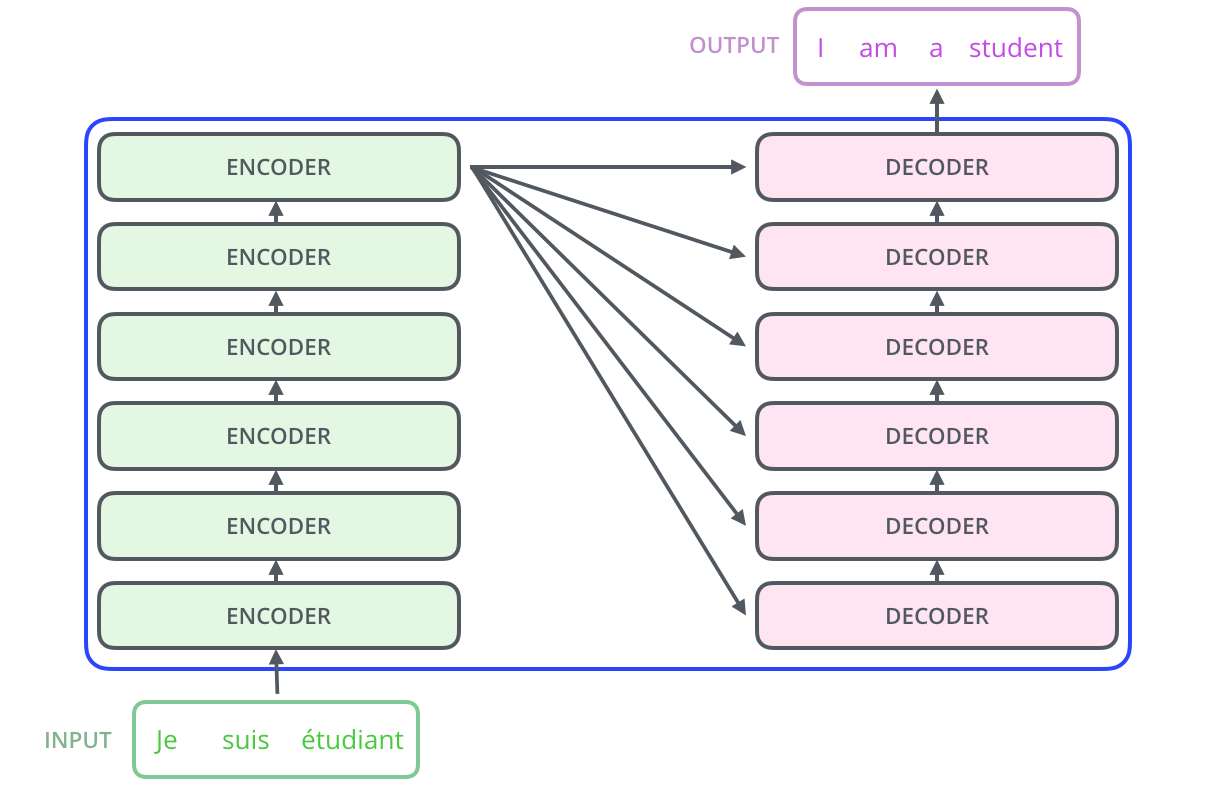## Positional Encoding

Transformer架构的一个特点就是用全连接层代替了RNN结构，以此大幅度提升训练的速度，但是用全连接层会丢弃位置信息，也就相当于将句子打散成词袋模型。为了弥补这个缺陷，位置信息编码就被引入了。一种方法是用不同频率的sine和cosine函数直接代入计算，另一种是学习出一份positional embedding。但其实两者的结果一样，那么更高效的第一种就被采用了。

\begin{aligned} PE_{(pos, 2i)} &= \sin(\frac{pos}{10000^{\frac{2i}{d_{model}}}}) \\ PE_{(pos, 2i+1)} &= \cos(\frac{pos}{10000^{\frac{2i}{d_{model}}}}) \end{aligned}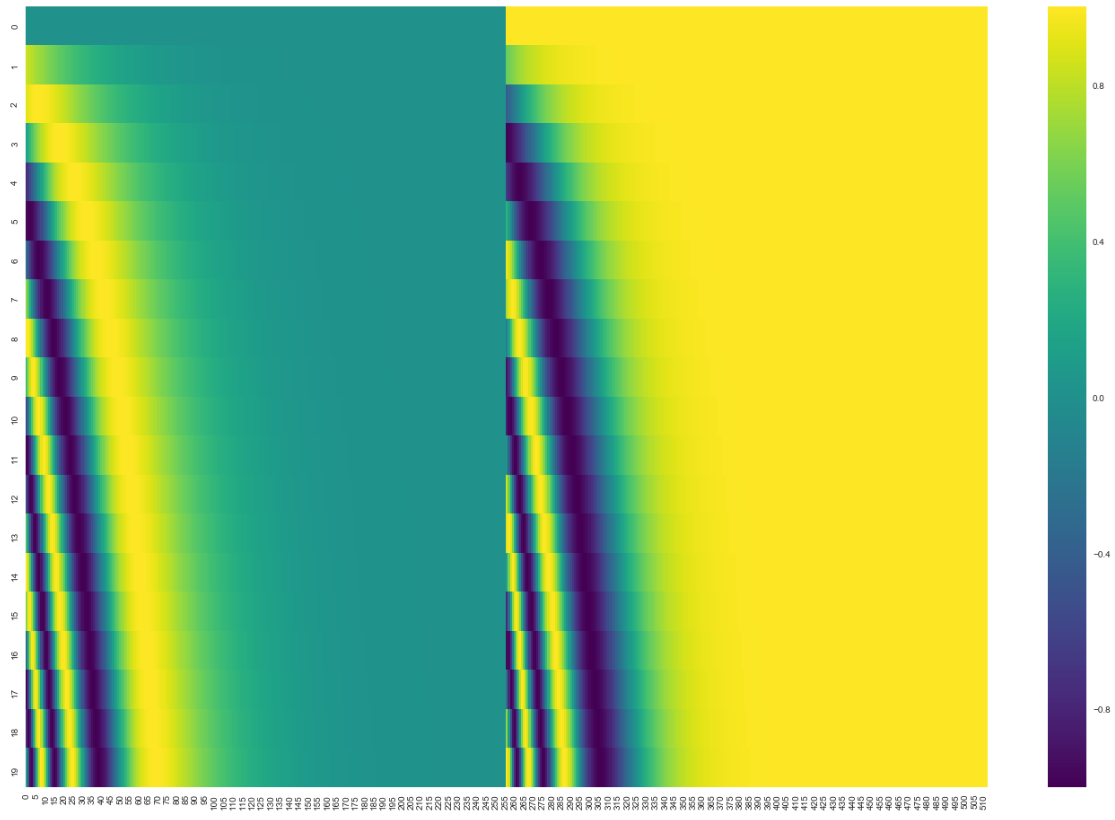Self-attention的作用是将每个输入分成Q（query）、K（key）、V（value）三部分，并完成下面的运算：

$Attention(Q, K, V) = softmax(\frac{QK^T}{\sqrt{d_k}})V$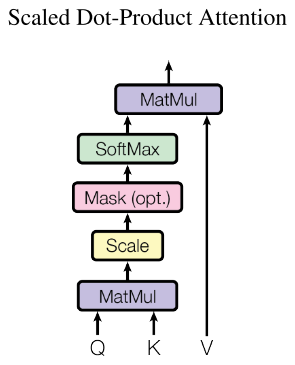\begin{aligned} head_i &= Attention(QW_i^Q, KW_i^K, VW_i^V) \\ MultiHead(Q, K, V) &= Concat(head_1, \dots, head_h)W \end{aligned}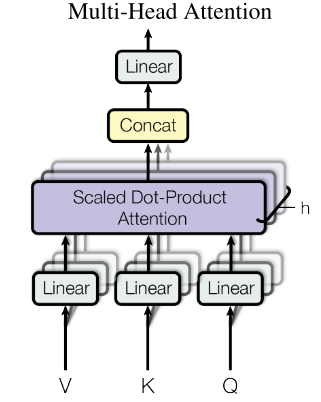## Fully connected feed forward

Transformer的不足之处：self-attention的计算复杂度为$O(n^2)$，当句子很长的时候，时间消耗相当大。另外长度为n的句子也并不一定需要全局attention，一些局部attention也能起作用，而局部attention甚至可以用CNN代替。

# ELMo（2018年2月）

ELMo是从深层双向语言模型中的 内部状态 学习而来的。首先定义双向语言模型的似然函数：

$\sum_{k=1}^N{\left( \log ⁡p(t_k |t_{1:k−1}; \Theta_x, \stackrel{\rightarrow}{\Theta}_{LSTM}, \Theta_s) + \log ⁡p(t_k |t_{k+1:N}; \Theta_x, \stackrel{\leftarrow}{\Theta}_{LSTM}, \Theta_s) \right)}$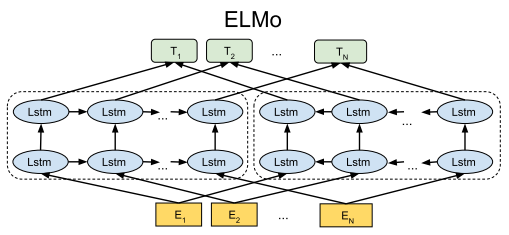$R_k = \{X^{LM}, \stackrel{\rightarrow}{h}_k^j, \stackrel{\leftarrow}{h}_k^j \}, j = 1, \dots, L$

\begin{aligned} s &= softmax(w) \\ E(R_k) &= \gamma \sum_{j=0}^{L}{s_j h_k^j} \end{aligned}

# BERT（2018年10月）

ELMo相比word2vec会有这么大的提升，可以看出预训练词向量的重要性。而BERT则是训练了一个龙骨级的语言模型，可以为下游任务提供词级、句子级的特征。

• 80%用[mask]替换
• 10%随机替换
• 10%不做替换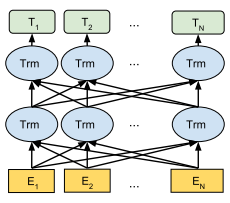BERT采用了“句子级负采样”，首先给定一个句子，它的下一句话为正例，而随机采样的另一个句子就是负例，将两个句子拼起来作为一个例子，然后在sentence-level做二分类。二分类的做法也是很脑洞的，因为考虑到Transformer可以无视空间距离做encoding，因此在句首添加了一个[cls]标记，让encoder对[cls]进行深度encoding，深度encoding的最高隐层即为整个句子对的表示。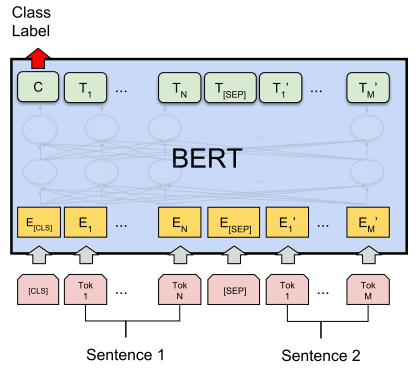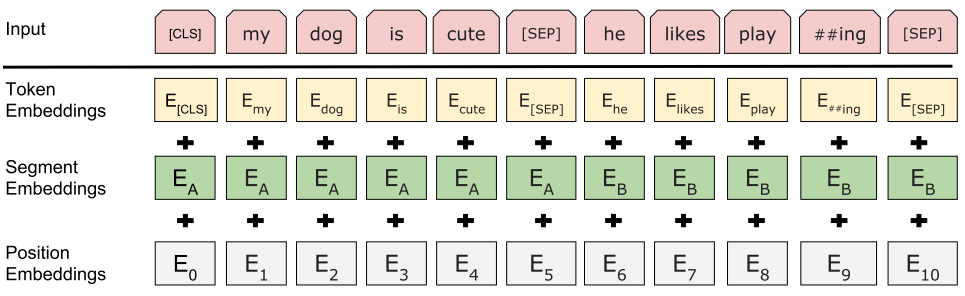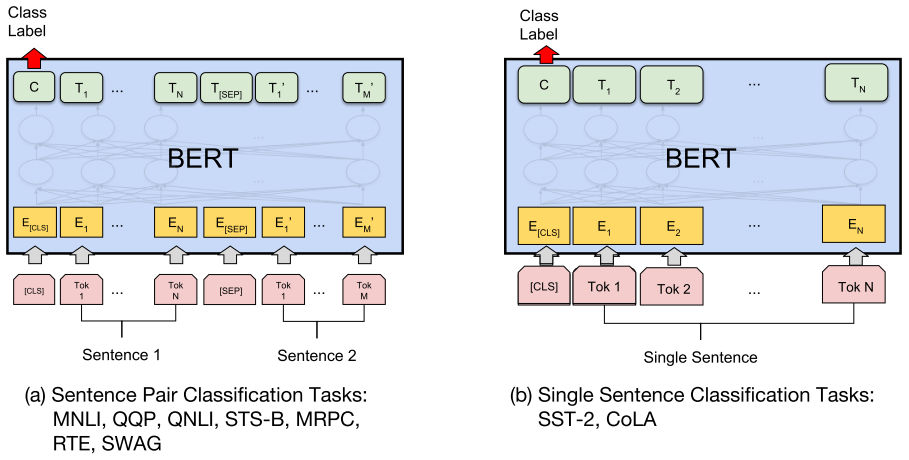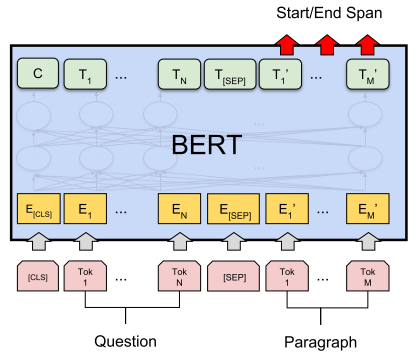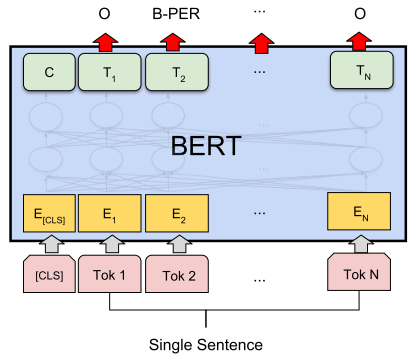# Transformer-XL（2019年1月）

Trm模型最大的缺点就是只能处理非常短的文本。而作为Trm的升级版，Trm-XL（XL是指extra long）在长距离依赖上表现十分好，并且在速度方面比Trm快1800多倍。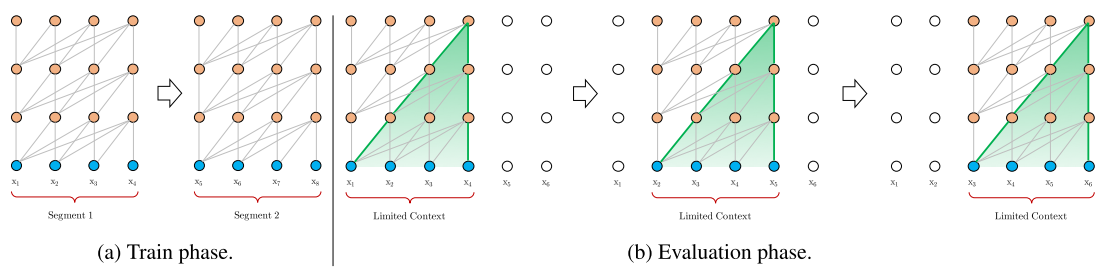Trm-XL在Vanilla Trm的基础上引入了两个创新点：

• 循环机制
• 相对位置编码

Trm-XL虽然和Vanilla Trm一样也会对文本分段建模，但是引入了段与段之间的循环机制，上一个chunk的隐含层信息被缓存起来，使得当前chunk在计算时能利用之前的信息。这样一来，Trm的边界被拓宽了，就以下面这张图为例，x12的信息能追溯至x3。另外，有了之前chunk的部分信息，下一个chunk就不需要重复计算这些联系，所以每次向前都是迭代一个chunk的大小，不再是龟速的每次一个step了。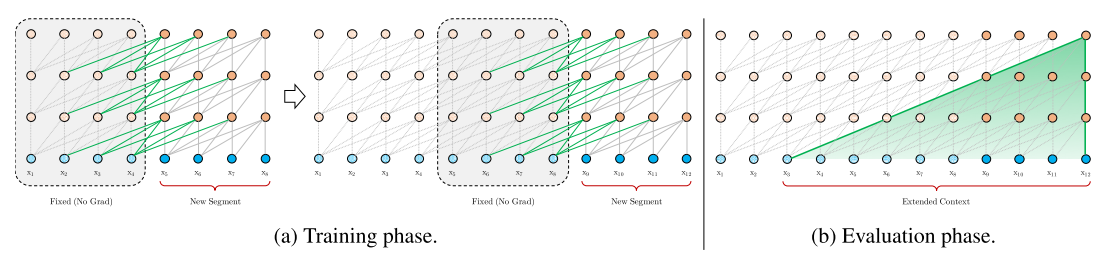Trm-XL的另一个改进是使用了相对位置编码的position embedding。对于同一个chunk中的询问q_i 和键k_j，他们之间的注意力分数可以分解为：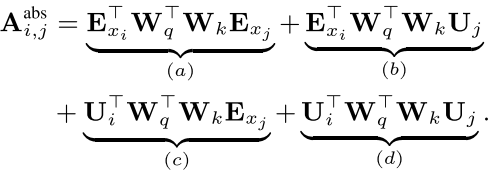$\left(W_q (E_{x_i} + U_i )\right)^T \left(W_k (E_{x_j} + U_j)\right)$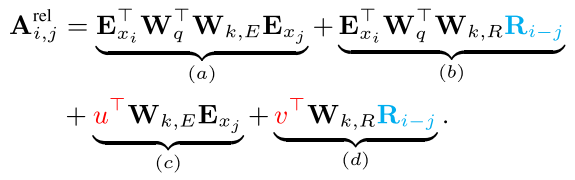# GPT-2（2019年2月）

OpenAI的GPT-2在写作上可谓是和人类难分真假，甚至有人想用GPT-2续写小说。那么这么强大的网络是怎么构造的呢？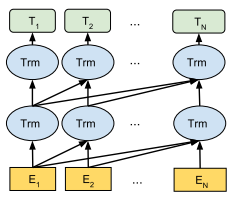$L_1 (U) = \sum_i{\log⁡ P(u_i |u_{i−k}, \dots, u_{i−1},\Theta)}$

$L_2(C) = \sum_{x,y}{log⁡P(y|x_1,…,x_m )} = \sum_{x,y}{\log⁡ softmax(h_l^m W_y)}$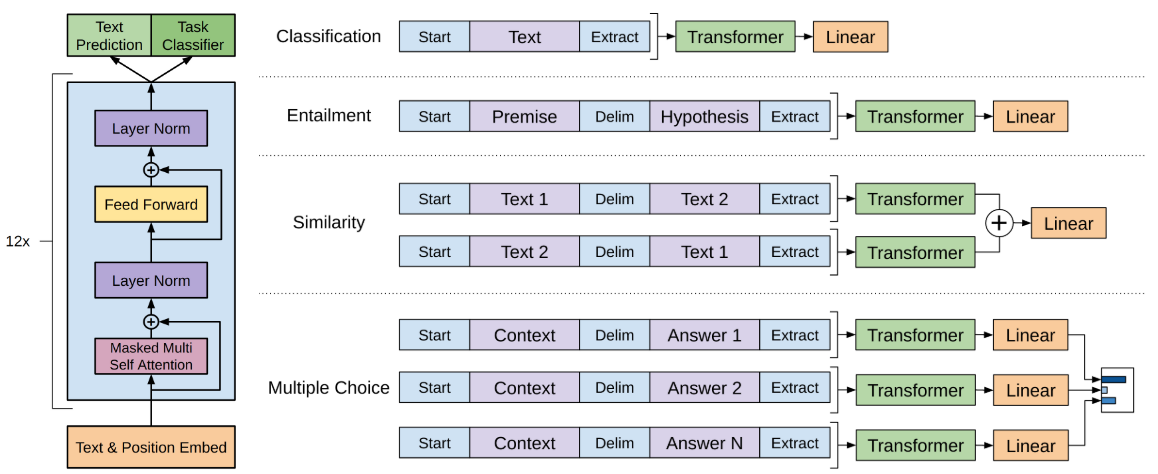$L_3 (C) = \lambda \times L_1(C) + L_2(C)$

GPT-2结构貌似和GPT相比没有太大的区别，就增加了Layer Normalization和权重初始化方式。

# XLNet（2019年6月）

## Permutation Language Model

$p_{\theta}(X_{z_t} = x | x_{z_{

$p_{\theta}(X_{z_t} = x | x_{z_{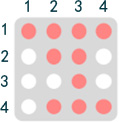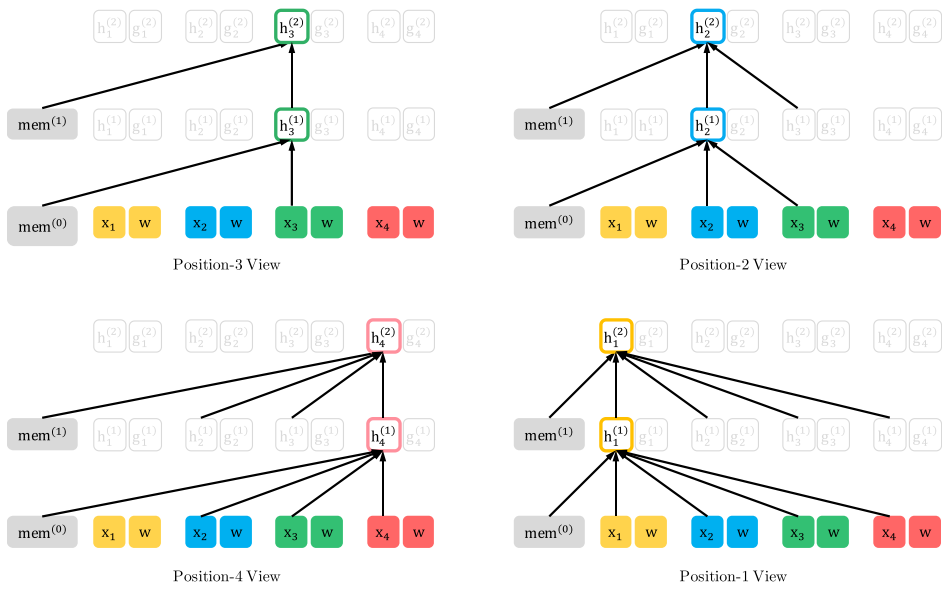## Two-stream Self-Attention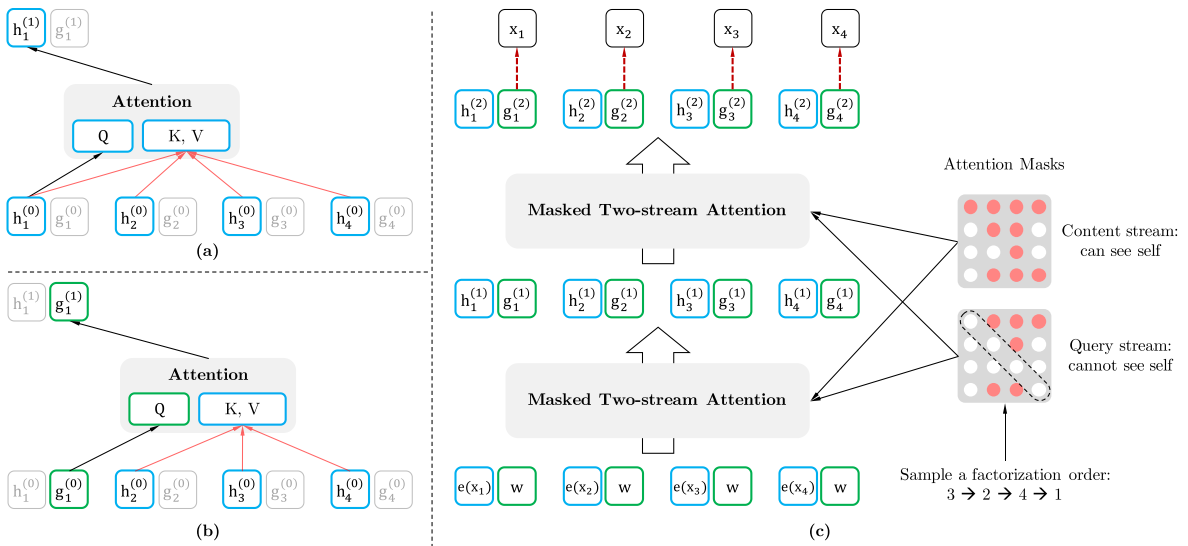$g_{z_t}^{(m)} = Attention\left( Q=g_{z_t}^{(m-1)},KV=h_{Z_{

$h_{z_t}^{(m)} = Attention\left( Q=h_{z_t}^{(m-1)},KV=h_{Z_{\le t}}^{(m-1)} \right)$

# 参考

## Word2Vec

• Mikolov, T., Chen, K., Corrado, G., & Dean, J. (2013). Efficient estimation of word representations in vector space. 1st International Conference on Learning Representations, ICLR 2013 - Workshop Track Proceedings, 1–12.

## GloVe

• Pennington, Jeffrey, Richard, S., & Christopher, M. (2014). GloVe: Global Vectors for Word Representation. Proceedings of the 2014 Conference on Empirical Methods in Natural Language Processing (EMNLP). 2014., 1532–1543. Retrieved from https://nlp.stanford.edu/pubs/glove.pdf
• 理解GloVe模型（Global vectors for word representation）

## FastText

• Joulin, A., Grave, E., Bojanowski, P., & Mikolov, T. (2017). Bag of tricks for efficient text classification. 15th Conference of the European Chapter of the Association for Computational Linguistics, EACL 2017 - Proceedings of Conference, 2, 427–431. https://doi.org/10.18653/v1/e17-2068
• Bojanowski, P., Grave, E., Joulin, A., & Mikolov, T. (2017). Enriching Word Vectors with Subword Information. Transactions of the Association for Computational Linguistics, 5, 135–146. https://doi.org/10.1162/tacl_a_00051
• Joulin, A., Grave, E., Bojanowski, P., Douze, M., Jégou, H., & Mikolov, T. (2016). FastText.zip: Compressing text classification models. 1–13. Retrieved from http://arxiv.org/abs/1612.03651
• 玩转Fasttext

## Transformer

• Vaswani, A., Shazeer, N., Parmar, N., Uszkoreit, J., Jones, L., Gomez, A. N., … Polosukhin, I. (2017). Attention is all you need. Advances in Neural Information Processing Systems, 2017-Decem(Nips), 5999–6009.
• 图解什么是 Transformer

## ELMo

• Peters, M., Neumann, M., Iyyer, M., Gardner, M., Clark, C., Lee, K., & Zettlemoyer, L. (2018). Deep Contextualized Word Representations. 2227–2237. https://doi.org/10.18653/v1/n18-1202

## GPT

• Radford, A., & Salimans, T. (2018). Improving Language Understanding by Generative Pre-Training. OpenAI, 1–12. Retrieved from https://s3-us-west-2.amazonaws.com/openai-assets/research-covers/language-unsupervised/language_understanding_paper.pdf
• Radford, A., Wu, J., Child, R., Luan, D., Amodei, D., & Sutskever, I. (2018). Language Models are Unsupervised Multitask Learners.
• NLP的游戏规则从此改写？从word2vec, ELMo到BERT

## Transformer-XL

• Dai, Z., Yang, Z., Yang, Y., Carbonell, J., Le, Q., & Salakhutdinov, R. (2019). Transformer-XL: Attentive Language Models beyond a Fixed-Length Context. 2978–2988. https://doi.org/10.18653/v1/p19-1285
• Transformer-XL解读（论文 + PyTorch源码）

## XLNet

• Yang, Z., Dai, Z., Yang, Y., Carbonell, J., Salakhutdinov, R., & Le, Q. V. (2019). XLNet: Generalized Autoregressive Pretraining for Language Understanding. 1–18. Retrieved from http://arxiv.org/abs/1906.08237
• XLNet:运行机制及和Bert的异同比较

## 其他

©️2019 CSDN 皮肤主题: 精致技术 设计师: CSDN官方博客